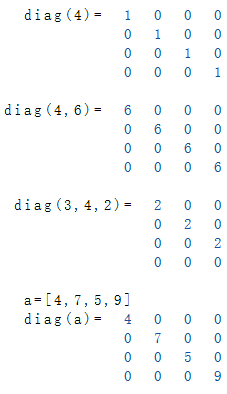# Diag Function

Generate a matrix with initialized diagonal

## Description

The Function $$Diag$$ generates a matrix of the specified size whose first diagonal is initialized with a real value.

If only one parameter is specified for the size of the matrix, the fields of the first diagonal are initialized with 1. Optionally, a value can be specified, which is to be used.

When a list is passed as a parameter, \ (Diag \) generates a matrix whose number of columns and rows equals the length of the list. The diagonal is initialized with the values in the list.

### Syntax

 Diag (a) Diag (a, v) Diag (a, b, v) Diag ([a..n]) size a x a a x a a x b len x len content The fields of the first diagonal contain a 1 The fields of the first diagonal contain the value v The fields of the first diagonal contain the value v The fields of the first diagonal contain the value of the argument

### Examples